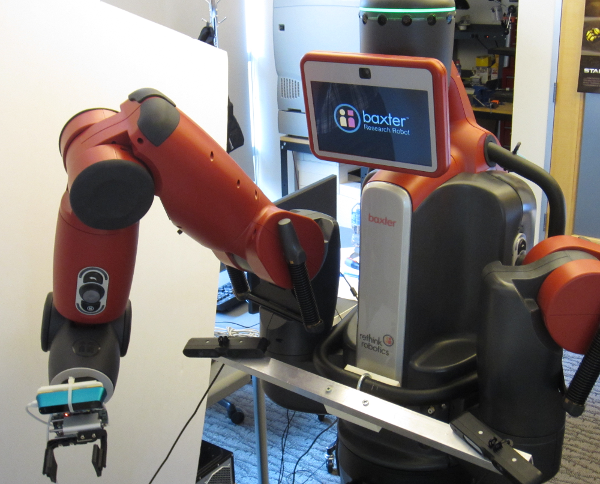## Brief Course Description

This course will introduce you to many of the foundational planning, control, and perception algorithms in robotics through the lens of robotic manipulation. You will implement each of these algorithms in a simplified context in Matlab. Then, you'll implement something more realistic in OpenRAVE, a simulation and planning toolchain used in the robotics manipulation research community. The focus will be on understanding and applying the ideas in new ways. There will be an end-of-semester project where you can show off what you've learned.

We will study most of the following topics (subject to change; see schedule tab above):

1. Kinematics/zero-order control
• Representation of rotation
• Manipulator forward kinematics
• Manipulator differential kinematics
• Cartesian end-effector control
2. Planning, Control
• Sample-based planning methods (RRT, PRM)
• Trajectory optimization methods
• Markov Decision Processes
• Linear optimal control (LQR, TVLQR)
3. Filtering, Localization, and Mapping
• Kalman filter, EKF
• SLAM algorithms
4. Computer Vision, Perception in Point Clouds
• RANSAC, ICP (iterative closest point)
• Linear filtering
• Object Detection Using Deep Learning

## Textbooks

The main textbook used in this course is Robotics, Vision, and Control: Fundamental Algorithms in Matlab by Peter Corke. You should buy this book. Additional material is taken from on-line sources that will be linked from the schedule page.

## Prerequisites

There are two informal pre-requisites for this course: 1) a working knowledge of linear algebra and 2) the ability to program in Python and Matlab. If you need a linear algebra refresher, have a look at the Khan Academy lecture links below:

Vector intro for linear algebra
Introduction to the matrix
Multiplying matrices
Introduction to the identity matrix
How to transform vectors using a transformation matrix
Introduction to eigenvalues and eigenvectors

Cheating and other acts of academic dishonesty will be referred to OSCCR (office of student conduct and conflict resolution). See this link.

## Lateness Policy

Late assignments will be penalized by 10% for each day late. For example, if you turned in a perfect homework assignment two days late, you would receive an 80% instead of 100%.

## Instruction Staff

Primary Instructor: Robert Platt ( r [dot] platt [at] neu [dot] edu )
Office hours: Tuesdays, 2-3pm, 526 ISEC, or by Appt.

TA: Yang Xia, xia.yang@husky.neu.edu
Office hours: Wedsnesdays and Fridays, 10am -- noon, 090 ISEC## Announcements

The Piazza page for this course is here.

Required course work includes:

• For those enrolled in CS5335, the projects will be graded to a higher standard than for those enrolled in CS4610.

## MATLAB assignments

There will be approximately 4 Matlab programming assignments using Peter Corke's Matlab Robotics Toolbox that will give you a chance to play with the algorithms in a Matlab environment.

## Directed Projects

Each student much complete one directed project that will involve creating robotic manipulation behaviors using OpenRAVE. OpenRAVE is a robotics toolchain that we often use in our research. The goal of these assignments is to introduce you to a realistic application of the ideas you will learn in this course. It will be due in early November. The amount of work should approximately be equal to two of the MATLAB homework assignments. All students may work in pairs. The projects of students in CS5335 will be graded to a higher standard than those of students in CS4610.

## Use of MATLAB and OpenRAVE in the course

You will be required to use MATLAB in conjunction with Peter Corke's Robotics Toolbox and Machine Vision Toolbox.

You will also be required to use OpenRAVE. It works best on Ubuntu, but it *should* also work on Windows. If you're good, you might also be able to get it working on Mac OSX. You'll need Python 2.7.